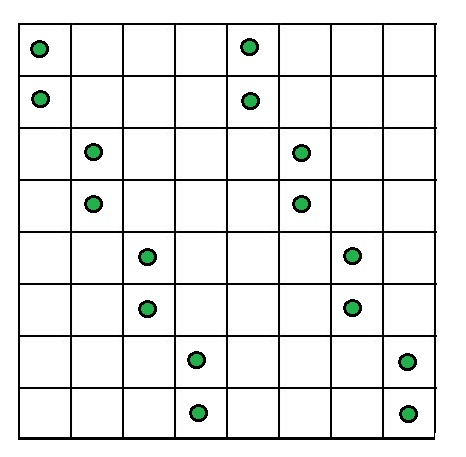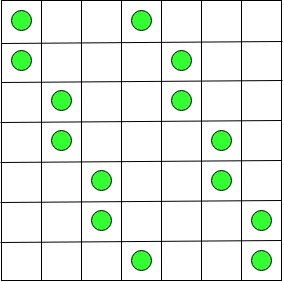Skip to content
Related Articles
Puzzle | The Counters and Board
• Difficulty Level : Medium
• Last Updated : 12 Dec, 2018

Given 2n counters where n > 1. You have to place these 2n counters on an n × n board so that no more than
two counters are in the same row, column, or diagonal.

Solution :
Since we need to place 2n counters into n rows and n columns of the given n x n board, with the condition that there can be at most two counters in the same row or in the same column. From this , we can conclude that exactly two counters have to be placed in each row and column.

Case 1 : For even n = 2k, we can obtain a solution by identical placement of n counters in the first k columns and the last k columns as explained below.
Let us assume that rows and columns of the board are numbered top to bottom and left to right, respectively.
Place two counters in the first two rows of columns 1 and k + 1, two counters in rows 3 and 4 of columns 2 and k + 2, and and so on, until finally counters are placed in rows n – 1 and n of columns k and 2k. Let us understand this with the help of an example when n = 8 :2n Counters problem for n = 8

Case 2 : For odd n = 2k + 1, k > 0, we can obtain a solution by placing two counters in rows 1 and 2 of column 1, two counters in rows 3 and 4 of column 2, and so on until counters are placed in rows n – 2 and n – 1 of column k. Then two counters are placed in the first and last rows of column k + 1. After that, k counters are placed in the right part of the board symmetrically with respect to the board’s central square to those in the left part: two counters are placed in rows 2 and 3 of column k + 2, in rows 4 and 5 of column k + 3, and so on, until rows n – 1 and n of the last column.Let us understand this with the help of an example when n = 7 :2n countern problem for n = 7

My Personal Notes arrow_drop_up